+ +

# 11. Back calculation of Effective Lens Position (ELP)

The position of the IOL in the pseudophakic eye influences the refractive outcome of cataract surgery, and the different methods used for predicting the « Effective Lens Position » (ELP) are the main milestones in the evolution of biometric IOL power calculation formulas. The rise of artificial intelligence techniques offers an additional opportunity to improve the quality of this prediction. This page is dedicated to the back-calculation of the position of the IOL considered as a thick lens, which is an essential part of developing an IOL power calculation formula that uses an optical model of a pseudophakic eye. At the end of this, an interactive spreadsheet allows simulations for this ELP back-calculation.

## Preliminary remarks

When a paraxial or ray-tracing-based formula provides intraocular lens power after the surgeon’s request, it assumes that the IOL will occupy a precise position in the anterior segment of the eye. Once this position is predicted, there is, in principle, only one IOL power possible to achieve the desired target refraction (at least with a thin lens paraxial eye model).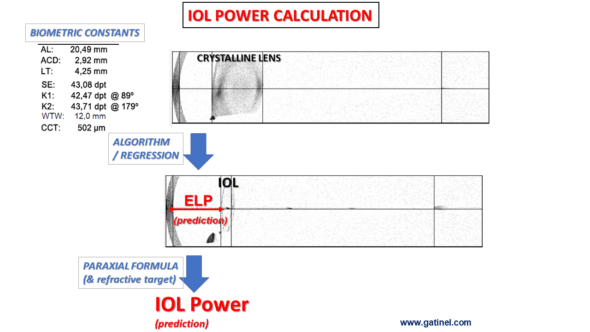This assumption regarding the IOL position is based on a predictive model that results from learning which one carried out thanks to retrospective data, such as postoperative refractions measured on many pseudophakic eyes whose preoperative biometric characteristics type and optical power of the inserted IOL are known. Whatever technique is used (multiple regression, AI algorithms), it is necessary to have a volume of well-documented data that is sufficient (sample size) and high quality.

Ideally, for every eye in the training set, we need preoperative data, the type and power of the implant placed, the refraction achieved after the cataract intervention, and a method to determine the physical position occupied the IOL in the anterior segment. The most direct method would obviously consist of measuring the implant postoperatively’s anatomical position (this position would be given by the depth of the anterior chamber of the pseudophakic eye, which can be measured by a recent biometer instrument or an OCT imaging device). This kind of data is unfortunately not documented routinely after cataract surgery.

It is, however, possible to back-calculate an estimated IOL position from the postoperative refraction, preoperative biometric data, and the power of the inserted implant. We understand intuitively that this computational approach provides information on a « functional » (or « optical ») position, and we will see that this is of particular importance to distinguish between a thin lens vs. a thick pseudophakic eye model.

In the present case, this model is built with thick lenses: this implies certain considerations regarding the concept of the « position of the IOL, » which we will detail in the next paragraph.

## IOL position in a pseudophakic eye model

In a thick lens pseudophakic eye model, the thick effective lens position (ELPT) depends on the physical position of the IOL in the eye,  the corneal thickness, and the IOL geometry that varies between IOL designs and power. This is because, using a thick lens pseudophakic eye, the physical distance between the cornea and the IOL (which is valid for a thin lens model) is replaced by the distance between the corneal principal image plane and the IOL principal object plane.  (more about the principal planes)

Actual lens position (ALP) has been defined as the physical distance measured from the anterior corneal surface to the anterior IOL surface. We can also define the internal lens position (ILP) as the physical distance between the posterior corneal surface and the anterior IOL surface. This value is independent of corneal thickness and IOL design (power distribution between the two surfaces of the IOL).

Using a formula for back-calculating the thick IOL ELPT in each eye of a training data set, one could generate data for training purposes for a machine learning algorithm or regression calculus. In addition to a well-documented pseudophakic eyes dataset (one must document preoperative biometry data, postoperative refraction, power, and IOL model), we also need a formula that allows back-calculation of the physical IOL’s position from these elements. This algorithm would then aim to predict the ILP  of eyes operated on for cataracts from their preoperative ocular biometry data, allowing the suitable IOL power to be chosen for the desired refraction using a paraxial optical formula ray tracing, or IA algorithm.

Within the IOL power calculation framework, we seek to develop a function predicting the value of the ILP for a given eye before cataract surgery. Once the value of the ILP is predicted from this function, we can predict the theoretical position of the principal object plane of the IOL that we plan to insert. In this case, it is assumed that the anterior surface of this IOL does not depend on its power and will be located at the ILP distance from the posterior surface of the cornea.

It is then possible to calculate the power of the IOL, which, positioned in this way, will make it possible to obtain the desired postoperative refraction. One can do this analytically by solving an equation of which the unknown is the IOL’s power or by performing an iterative calculation, i.e., by « trying » several powers of IOL up to achieve the desired target refraction. The assumption made here is whatever the power applied. IOL model, the anterior surface of the IOL would be positioned identically (at the same ILP): we can, of course, make a slightly different assumption that takes into account the thickness of the implant and thus back-calculate the position of the median plane of the IOL to insert.

Whatever method is used to determine the implant’s physical position relative to the cornea’s posterior surface, it is first necessary to back-calculate the value of the ELPT using a thick lens paraxial model and it is on this point that we will focus our efforts.

## Thick lens paraxial model for the pseudophakic eye

The schematic eye is represented as an optical system combining the cornea and the IOL, with each ‘lens’ being defined by its anterior and posterior radius of curvature, its refractive index, and its thickness.

In our model, the refractive index of the vitreous is not equated to the refractive index of the aqueous.

The design of the IOL (its anterior and posterior radii) can vary for the same paraxial power depending on the Coddington shape factor. Once obtained, the value of the ELPT can be used to compute the anatomical lens position (ALP), which corresponds to the distance separating the anterior corneal and IOL vertices.

The internal lens position (ILP) equals the ALP minus the central corneal thickness.

Using the properties of such a thick lens paraxial eye model, we determined the following formula:Where:

• ALT is equal to the anatomical axial length of the emmetropic eye (ALA) reduced by the distance between the principal planes of the implant (HiH’i) and the distance between the anterior surface of the cornea and the secondary principal plane of the cornea (S1H’c).
• ELPT is referred to here as the effective lens position of a thick IOL, i.e., the distance separating the cornea’s principal image plane from the IOL’s principal object plane (H’cHi).
• na is the refractive index of the aqueous humor
• nv is the refractive index of the vitreous
• Dc is the total power of the cornea
• Di is the total power of the IOL

## Determination of the ELPT

One can solve the preceding equation for ELPT :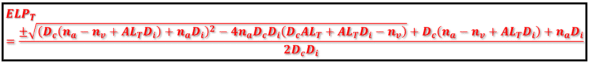The ± sign must be replaced by – when Di>0 (IOL power is positive) and by + when Di<0 (IOL power is negative).

This equation looks complicated but can be easily programmed in a spreadsheet. It provides the value of the ELPT in an emmetropic pseudophakic eye.

The ELPT value can be computed for a non-emmetropic pseudophakic eye after replacing Dc by Dce in the preceding equation,  where Dce is the sum of Dc and the vergence in the corneal plane of a spectacle lens of power equal to SE placed at distance d from the corneal vertex (neglecting the distance S1Hc).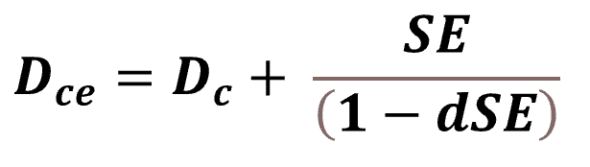This amounts to giving the cornea emmetropizing power so that one can use the ELPT resolution equation.

NB: The ELPT resolution equation could be used to determine the effective thin lens position, denoted ELPt, obtained in a thin lens model where the cornea and IOL have a null thickness (S1S2=S3S4=0) and  ALA=ALT. Conversely, when we use this formula in a thick lens context, ELPT corresponds to a distance between principal planes, which depends not only on the anatomical position of the IOL to the cornea but also on the geometry of the IOL (ratio between the anterior and posterior curvatures, central thickness).

From the value of the ELPT, we can obtain the value of the ILP, which (we assume) does not depend on the power of the implant placed.

The relationship between the thick lens position ELPT and the anatomical lens position of the IOL  (ALP for anterior lens position) is expressed as follows:As we know the characteristics of the cornea thanks to the preoperative biometry and those of the inserted IOL, we can calculate the value of these segments to obtain the ALP.

Finally, the internal lens position (ILP: distance between the vertex of the corneal posterior surface and the  anterior surface of the IOL) is given by: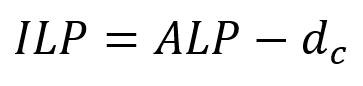where dc is the central corneal thickness (S1S2).

## Computation of the  ELPT, ALP, and ILP

The following spreadsheet calculates the effective position-related variables of the thick IOL in a pseudophakic eye from biometric data, postoperative refraction, and IOL geometry (Coddigton shape factor). Rca and Rcp are the anterior and posterior corneal surface radii, respectively. di is the IOL central thickness. Ria and Rip are the anterior and posterior IOL surface radii, respectively, computed from the IOL power and Coddigton values.

By varying certain parameters, we can indirectly analyze the influence of the position of the implant on postoperative refraction (due to the apparent complexity of the equation that provides the value of the ELPT, it is difficult to predict the influence of a particular parameter on it). If we reduce the value of the power of the anterior face of the cornea (by increasing the value of the radius of curvature Rca), we observe a reduction in the value of the ILP (the back-calculated position of the IOL is more anterior). We can also directly appreciate the influence of design through variations of the Coddington factor, which causes a displacement of the principal planes of the IOL (while the physical position of the latter does not vary).

We have published a paper focused on the ELP back calculation.

This data-inferred approximation of the physical position of the IOL in the eye could increase IOL calculation accuracy. As demonstrated concretely with this spreadsheet, the  ILP can be calculated for well-documented eyes comprising a training set and then used as the designated target to predict using an algorithm (or regression task), with the preoperative biometric +/- demographic (age, sex, etc.) parameters as features.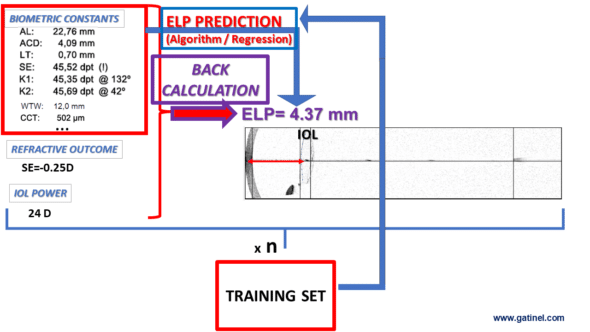For example, multiple linear regression can be used, with axial length, the anterior radius of curvature, preoperative aqueous chamber depth, lens thickness, central corneal thickness, and white-to-white diameter as independent variables.

### Une réponse à “11. Back calculation of Effective Lens Position (ELP)”

1.Raanan Shabtai dit :

Hi. Merci pour le tableux excel.
le parametre « Vertex distance (m) » a un regle incorrect. 0-0.002 -devois etre 0-0.02
:-)

Merci!

Warning: error_log(/home/clients/0e98f30bad73cbe357ff222deca9aba0/web/gatinel/wp-content/mc_data/53c4b42756475b28ee65f2b1a786a741-mc.log): failed to open stream: Disk quota exceeded in /home/clients/0e98f30bad73cbe357ff222deca9aba0/web/gatinel/wp-content/plugins/wpremote/protect/logger/fs.php on line 16